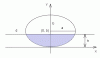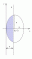I have forgotten

Area

Edge Triangle

Computes the area of a trapezium within a triangle with a fixed edge.
 double edge_triangle (double a, double b, double c, double h)[inline]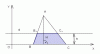Circle

Computes the area of the circular segment within a circle tangent to a reference line.
 double circle (double r, double h)[inline]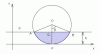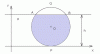Ellipse

Computes the area of the ellipse segment determined by the intersection of an ellipse and a line parallel to the reference line.
 double ellipse (double a, double b, double h)[inline]